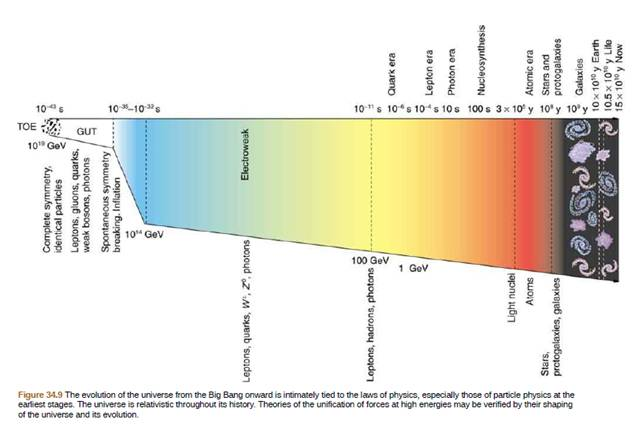Chapter 34, Problem 11CQ

Chapter
Section
Textbook Problem

If the smallest meaningful time interval is greater than zero, will the lines in Figure 34.9 ever meet?To determine

Whether the lines will ever meet or not as shown in Figure 1, if the smallest meaningful time interval is greater than zero.

Explanation

Introduction:

Smallest meaningful time interval is defined as the time taken by the light to travel the planks distance and it is in order of decimal order of seconds.

The Given figure is shown below.

Still sussing out bartleby?

Check out a sample textbook solution.

See a sample solution

The Solution to Your Study Problems

Bartleby provides explanations to thousands of textbook problems written by our experts, many with advanced degrees!

Get Started

Find more solutions based on key concepts